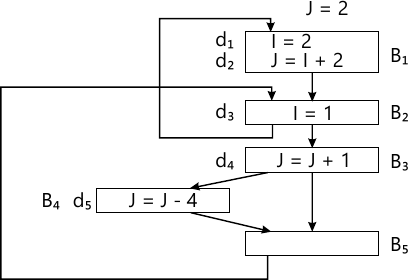MORE IN Principles of Modern Compiler Design
SPPU Computer Engineering (Semester 7)
Principles of Modern Compiler Design
December 2014
Total marks: --
Total time: --
INSTRUCTIONS
(1) Assume appropriate data and state your reasons
(2) Marks are given to the right of every question
(3) Draw neat diagrams wherever necessary

Answer any one question from Q1 and Q2
1 (a) Explain the interaction between Lexical Analyzer and Parser in first pass of compiler.
4 M
1 (b) Generate predictive parsing table for the given grammar and parse the string acbbgf.
S → a B D h
B → c C
C → b C/E
D → E F
E → g/E
F → f/F
10 M
1 (c) Differentiate between SLR, LR(K), and LAIR parsers.
4 M

2 (a) Differentiate between top-down and bottom-up parsers.
4 M
2 (b) Generate SLR parsing table for the given grammar and parse the string id1+id2+id3*id4
E → E+T / T
T → T * F / F
F → id
8 M
2 (c) Explain: operator Precedence parser.
6 M

Answer any one question from Q3 and Q4
3 (a) Differentiate between S-attributed and L-attributed grammar.
4 M
3 (b) What is syntax tree? Give YACC specification to generate syntax tree for expression a+b*c.
8 M
3 (c) Explain: static & Dynamic checking of types.
4 M

4 (a) Discuss working of Recursive - Descent parser with suitable example.
6 M
4 (b) What is type checking? Give various type expressions.
6 M
4 (c) Explain in brief: syntax directed translation.
4 M

Answer any one question from Q5 and Q6
5 (a) Explain how boolean expressions are evaluated while generating intermediate code. Explain use of marker non-terminals and backpatching.
6 M
5 (b) Given code: a = b * c + d
write syntax directed translation scheme to translate above code into postfix notation.
6 M
5 (c) Explain: Need for Intermediate code
4 M

6 (a) Generate 3-addr code for following statements. specify the translation scheme used.
A[i] = B [i] + C
P = A [i]
8 M
6 (b) Compare: Quadruple, Triple, Indirect Triple
6 M
6 (c) Generate Triple representation for following:
A = B * (C + D) / E
2 M

Answer any one question from Q7 and Q8
7 (a) Explain: Source Language issues
4 M
7 (b) Explain following
Call by Value
Call by Name
Call by Reference
6 M
7 (c) Discuss in detail the interaction of symbol table with various phases of compiler.
6 M

8 (a) Explain: Issue related to nested procedures.
4 M
8 (b) Explain run-time management of variable length data.
6 M
8 (c) Discuss storage organization and allocation strategies.
6 M

Answer any one question from Q9 and Q10
9 (a) What is DAG? Explain its use in code generation. Generate DAG:
T1 = A+B
T2 = C+D
T3 = E-T2
T4 = T1-T3
8 M
9 (b) What is need for next- use information? Explain how to compute next ? use information.
6 M
9 (c) Explain the concept of basic block.
2 M

10 (a) Explain: Issue in code generation.
6 M
10 (b) Determine cost of following instruction sequence. (Clearly mention your assumptions)
MOV b, Ro
MOV Ro, a
MOV * Rp * Ro
6 M
10 (c) What is peephole optimization?
4 M

Answer any one question from Q11 and Q12
11 (a) Which are basic dataflow properties? Explain in detail.
8 M
11 (b) Given flow graph:
Generate IN, OUT, GEN and KILL sets for all blocks.8 M
11 (c) What is control ? flow analysis?
2 M

12 (a) Explain following optimizations with examples:
i) Common Subexpression Elimination
ii) Code movement
iii) Variable Propagation
iv) Strength reduction
8 M
12 (b) Explain: Meet Over Path (MOP) solution to data flow problems.
4 M
12 (c) Explain algorithm for global common subexpression elimination. (Support your answer with example flowgraph).
6 M

More question papers from Principles of Modern Compiler Design